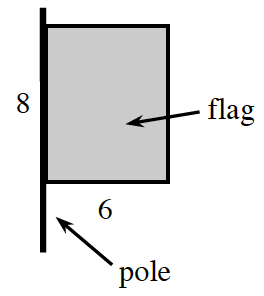Home > APCALC > Chapter 1 > Lesson 1.1.1 > Problem1-3

1-3.

In this course, a “flag” is defined as a geometric region attached to a line segment (its “pole”). An example is shown at right.

1. Imagine rotating the flag about its pole and describe the resulting three-dimensional figure. Draw a picture of this figure on your paper.

Pretend you are the pole that is $8$ ft tall. Hold out your ($6$ ft) arm
perpendicular to your body and spin around $360^\circ$. Imagine the shape.

The shape is a cylinder with height of $8$ and radius of $6$.

1. Calculate the volume of the rotated flag.

• $\text{volume of cyclinder = }\pi r^2h$

Use the eTool below to visualize the flag.
Click the link at right for the full version of the eTool: Calc 1-3 HW eTool